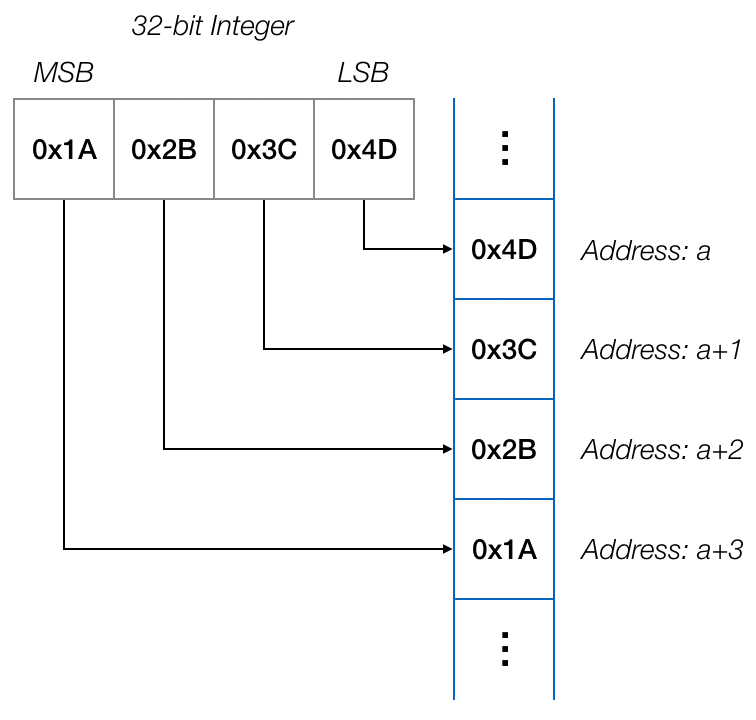# Endian-ness in Squirrel Code

### Understand The Ordering Of Multi-Byte Integers

Squirrel’s native integers are 32-bit signed values. The imp, like all ARM-based microcontrollers, is a ‘little-endian’ device. This means that the four bytes which are used to store each of Squirrel’s 32-bit integers contain the least-significant byte first (ie. at the lowest memory address of the four) and the most-significant byte last (at the highest memory address). This is illustrated in the following diagram:Generally, a Squirrel application need not worry about the order of the bytes in a word as this is handled behind the scenes. However, some applications do need to be aware that not all devices their host may be connected to store numbers in this way. These others are said to be ‘big-endian’. They store words in the opposite order to that used by the imp: the least-significant byte this time comes last (ie. at the highest memory address of the four) and the most-significant byte first (at the lowest memory address).

This means that when an application asks such a device for a word, that word will be transmitted to the imp as a series of bytes ordered according to its endian-ness. The application interpret this word value incorrectly if it doesn’t take into account endian-ness.

For example, in the above example, the value stored is (in decimal) 439041101. However, if we read the bytes in the reverse order (ie. reverse the number’s endian-ness), it becomes 1295788826.

Typically, such values will be read in from a bus — I²C, for example — as a sequence of bytes or characters which are initially written into a blob or a string, and then converted into an integer or a float so calculations can be performed with them. To convert correctly, you need to know the endian-ness of the data source, which will be indicated by the component’s datasheet.

Let’s say we are reading in an unsigned 16-bit (ie. two-byte) value. If the source is big-endian (MSB first) and the word is read into the blob or string buffer, then convert as follows:

``````local value = (buffer << 8) | buffer;
``````

This sets the value of buffer0 (the MSB) as bits 8 through 15 of value and buffer (the LSB) as bits 0 through 7.

If the value is little-endian (LSB first) then use:

``````local value = (buffer << 8) | buffer;
``````

You don’t have to order the bytes to match the imp’s endian-ness because Squirrel does this for you; you only need to make sure you’ve handled the incoming byte order correctly.

The process is slightly more complicated when you’re dealing with signed integers, as you need to subsequently ‘extend’ the 16-bit integer’s sign bit so that the 32-bit value sign bit is set to match:

``````// Big-endian
local value = (buffer << 8) | buffer;
value = (value << 16) >> 16;
``````
``````// Little-endian
local value = (buffer << 8) | buffer;
value = (value << 16) >> 16;
``````

The final line in each snippet shifts the 16-bit value up 16 bits so that the 32-bit integer sign bit (bit 31) is set. Then the initial value is returned to its correct position in the 32-bit sequence, preserving the sign bit in the process.